Sandra Cane

•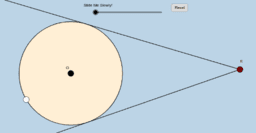Circumscribed Angle Theorem

Activity

Sandra Cane

•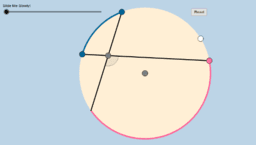Angles Inside the Circle Theorem

Activity

Sandra Cane

•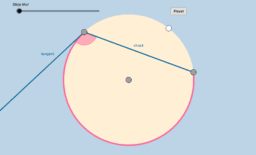Tangent and Intersected Chord Theorem

Activity

Sandra Cane

•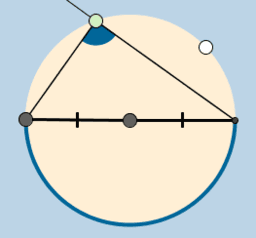Thales Theorem

Activity

Sandra Cane

•Geo Lesson 10.4 Inscribed Angles

Activity

Sandra Cane

•Lesson 2.6 Vertical Angles Congruence Theorem

Activity

Sandra Cane

•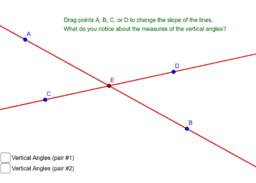Lesson 2.6 Linear Pair Postulate

Activity

Sandra Cane

•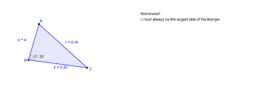Geometry Lesson 9.1 Pythagorean Inequality Theorem

Activity

Sandra Cane

•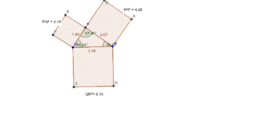Lesson 9.1 Pythagorean Inequalities Theorem

Activity

Sandra Cane

•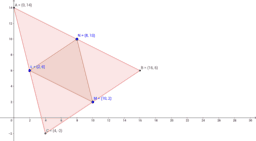Activity

Sandra Cane